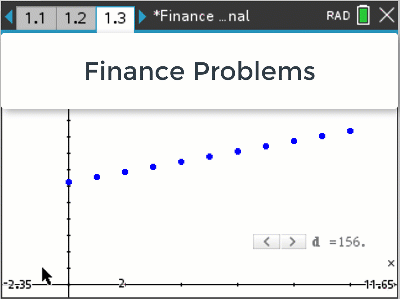# Activities

•• ##### AuthorAust Senior

50 Minutes

• ##### Device
•TI-Nspire™ CX CAS
•TI-Nspire™ CX
• ##### Software

TI-Nspire™ CAS
TI-Nspire™

5.0

## Finance Problems#### Activity Overview

What is the difference between simple and compound interest? Visually we can see that simple interest is linear and compound is non-linear. These visuals can be used to improve student understanding of what a significant difference this can make over time. Dynamic representations illustrate how increasing the number of compounding periods and interest rates dramatically effect the contribution compounding interest has to the overall balance. Recursive techniques, including spreadsheets and formulas help reinforce the concepts numerically. See how you can retire with a million dollars on just \$60.00 per week.

#### Objectives

This activity aims to create a greater understanding of the differences between simple and compound interest by providing powerful, interactive visuals, challenging questions and an engaging context: "Would you like to retire a millionaire?" Students also learn how to use spreadsheet formulas for recursion and modelling and compare these outcomes to basic formulas and the Finance Solver wizard.

#### Vocabulary

• Recursion
• Simple Interest
• Compound Interest
• Principal
• Future Value
• Payment
• Linear and non-Linear